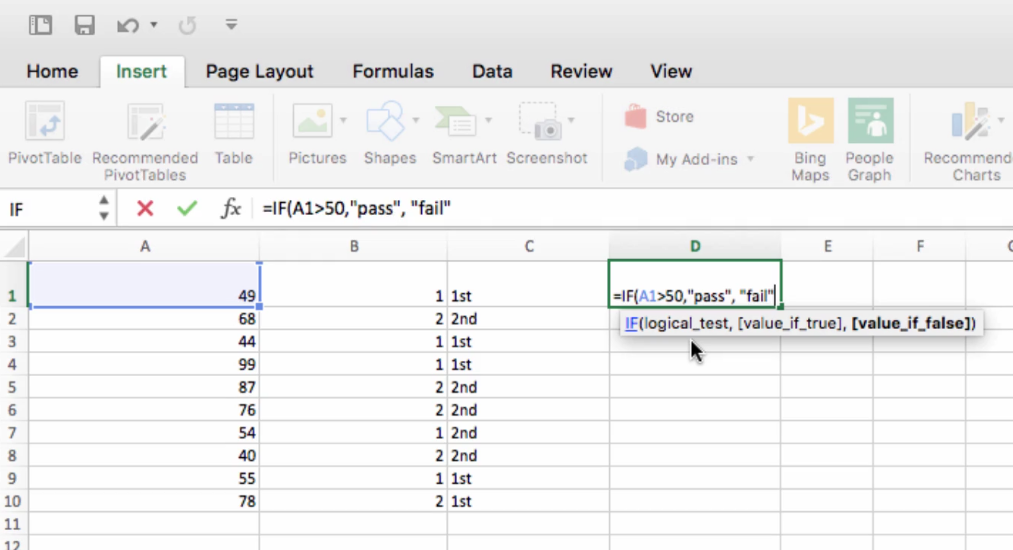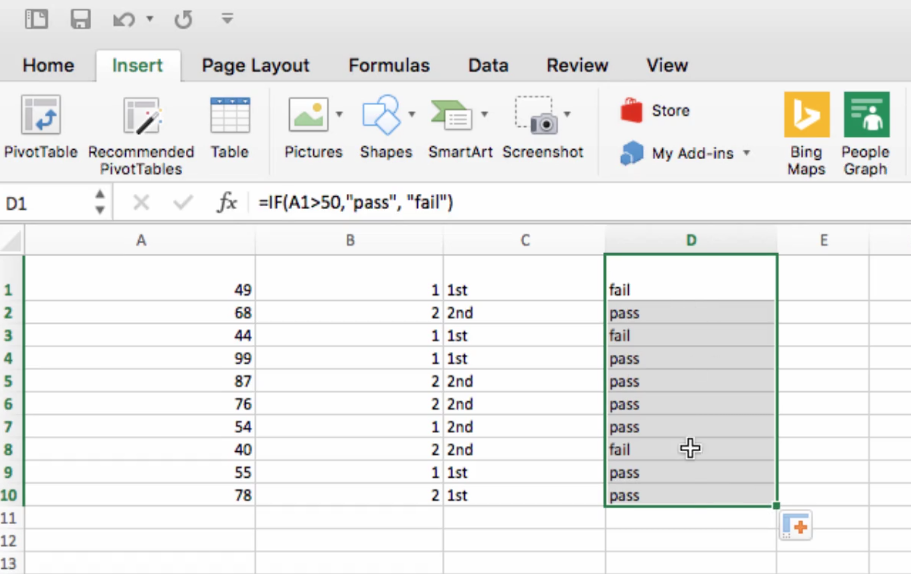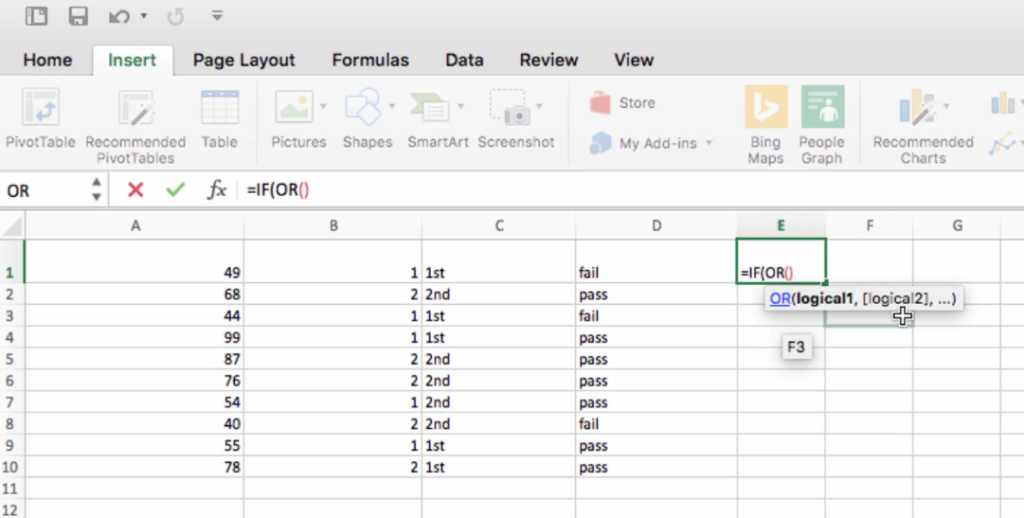## Popular Functions in Excel: Lesson 1 – "IF"## In this Excel miniseries, we’ll cover the most popular and commonly used functions in Microsoft Excel. This first lesson in our mini series will cover the “IF” function, which checks whether a condition is met and then returns different values based on whether it’s TRUE or FALSE.

Essentially, employing the IF command allows you to program a conditional function into Excel. IF the condition is true, you will get one value returned in the cell, and you will get a different value returned if the condition is false instead. If you’ve ever done any programming, you’ll be very familiar with these types of functions that employ a logic system to decide how they need to operate.

So let’s take a look at the basic breakdown of IF. Once you start the function, you’re going to need to enter three values into the parentheses.

The first is going to be the condition – this is the value that needs to be true or not, and its status is what will determine what value is returned.

Next, in brackets, you’re going to enter the value that should be returned from the cell if the condition is met, followed by a comma, followed by the value that you want returned if the condition is false. Then you can close the parenthesis.

Let’s look at a simple version of how this works. For example, you could use this function to organize students’ test scores by pass/fail.

Let’s say a student passes if he scores above 50 on his exam, but fails if his score is below 50. So we’re going to say if the value of cell A1 is greater than 50, we want to return the value “Pass,” and if it is less than 50, we want it to say “Fail.”Then, we could simply drag this formula down and have a pass/fail value indicated based on the number in the A column.You can also use other commands to expand the functioning of the IF command. For instance, you can use AND to create a set of conditions which must both be met in order for the True value to be returned.Another alternative is that you can insert an OR command inside of the IF function. So, in this case here, the value for a True condition will be met if either of these requirements are met.